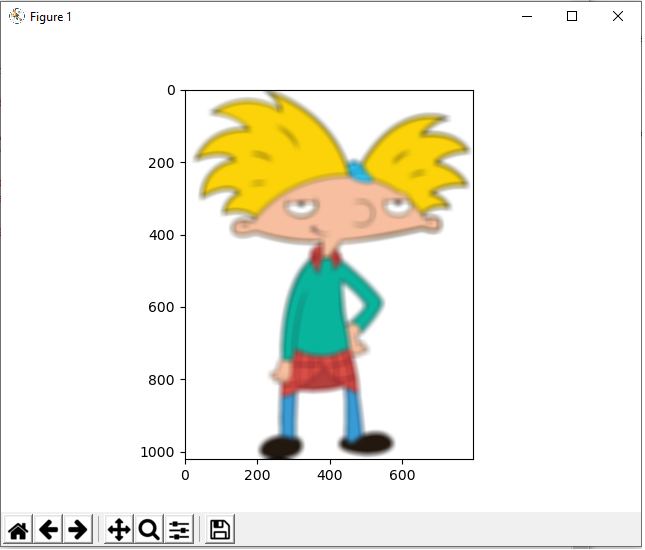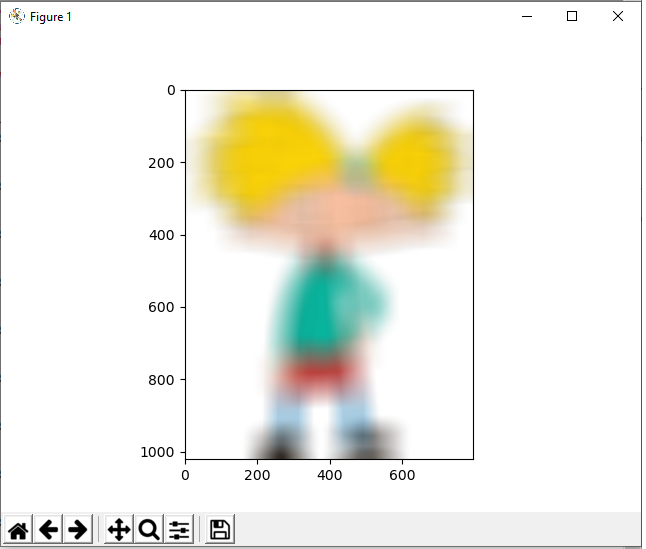﻿ How to Blur an Image in Python using OpenCV ﻿# How to Blur an Image in Python using OpenCVIn this article, we show how to blur an image in Python using the OpenCV module.

OpenCV allow us to perform a number of different image manipulations, including resizing images, adjusting the brightness of an image, as well as doing things such as blurring images.

At times, for different, you may want to blur an image.

This could be the case for something like a website that only shows image to those who are members and blurs certain images to those who aren't members.

Using OpenCV, we can easily blur images so that the distinct image cannot be seen but merely a blurred version of the image.

We blur images with OpenCV using the cv2.blur() function.

The cv2.blur() function takes in 2 parameters.

The first parameter is the image that we want to blur.

The second parameter is the the amount that we want to blur the image, which we specify with the ksize attribute. The greater the ksize value, the greater the image gets blurred.

So let's see how this works.

We will blur the following image of Arnold from the Nickelodeon show Hey Arnold seen below.Using the following code shown below, we will blur the image of Arnold.

Let's now go over this code.

First, we import all the modules we need which is cv2 (OpenCV) and matplotlib (to display the image).

Next we create a variable, img, which we use to read in our image .

We then create a blurred variable, which we will use to store our blurred image. We do this through the cv2.blur() function. Again, this will blur our image, img. We then specify the ksize to determine the degree of blurriness that we want. The higher the values, the greater the blurriness of the image. In this first example, we use a ksize of (20,20). You can play with these values, but we will show several in this article.

We then show the image using matplotlib functions.

This produces the following image shown below.Next we will adjust the ksize to blur the image greater.

Below we have all the same code only with a greater ksize attribute.

The ksize attribute of the cv2.blur() function is now (50,50).

This produces the following image shown below.Next we adjust the ksize to blur the image even greater than the previous example.

Below we have all the same code only with a greater ksize attribute.

The ksize attribute of the cv2.blur() function is now (100,100).

This produces the following image shown below.And this is how we can blur images in Python using the OpenCV module.

Related Resources